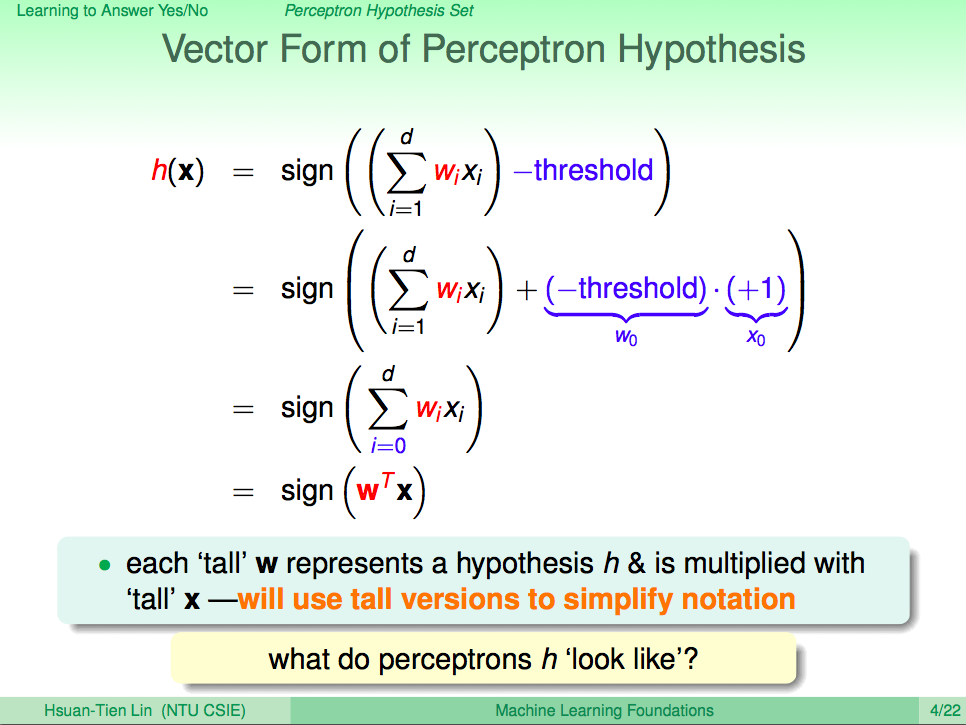# 機器學習基石-第二講筆記

## 感知學習演算法## 碰到的問題

### 如何調整權重 (w)

$$\lvert\overrightarrow w\rvert$$ 是 w 這個向量的長度，而 $$\theta$$ 則是 wx 之間的夾角。由於向量的長度不會為負數，因此影響 $$w^Tx$$ 是正還是負的主要因子就是 $$\theta$$ 了。 $$\cos(\theta)$$ 的特性是當 $$\theta$$ 在 $$0^\circ$$ 與 $$90^\circ$$ 之間時是正數，在 $$90^\circ$$ 與 $$180^\circ$$ 度之間時是負數。透過將 $$w_{t+1}$$ 設為 wx 兩個向量相加能夠縮小 $$w_{t+1}$$ 與 x 之間的夾角進而使結果趨於正數，反之亦然。而根據公式，這個該加還是該減就取決於 $$y_{n(t)}$$ 囉。

### 為什麼分割線是垂直於 wwx 的夾角 $$\theta$$ 小於 $$90^\circ$$ 時結果為正 (圖中的圈圈)，大於 $$90^\circ$$ 時結果為負 (圖中的叉叉)。因此與夾角垂直的線自然就成了正與負的分水嶺囉。

## Pocket Algorithm

PLA 要能解決問題必須有一個很大的前提，就是輸入資料能夠被完整的分成兩部分 (分黑即白)，不然 PLA 會不斷偵測到錯誤而無法停下。在資料無法被完整的二分化時，我們可以透過 Pocket Algorithm 來達到比較好的結果 (非完美) 同時能使演算法停止。Hello, I'm Rhadow. A software engineer curious about how nature works. Dreaming to simulate our world in computer one day.